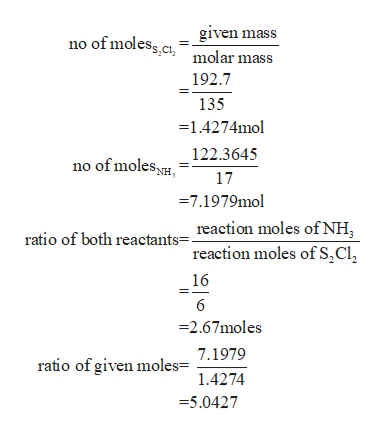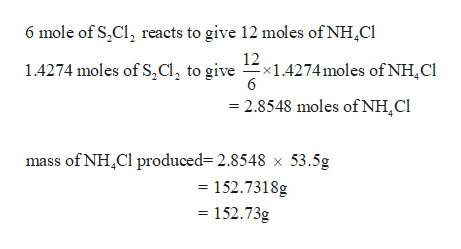# Consider the following reaction:6 S2Cl2 (l) + 16 NH3 (g) → S4N4 (s) + S8 (s) + 12 NH4Cl (s)What is the maximum mass of ammonium chloride that can be produced by the reaction of 192.7 g of disulfur dichloride and 122.36449999999999 g of ammonia?

Question
113 views

Consider the following reaction:

6 S2Cl2 (l) + 16 NH3 (g) → S4N4 (s) + S8 (s) + 12 NH4Cl (s)

What is the maximum mass of ammonium chloride that can be produced by the reaction of 192.7 g of disulfur dichloride and 122.36449999999999 g of ammonia?

check_circle

Step 1

Given data

Mass of disulphur dichloride= 192.7g

Mass of ammonia = 122.36449999999999g

= 122.3645g

Step 2

No of moleshelp_outlineImage Transcriptionclosegiven mass no of moless c molar mass 192.7 135 1.4274mol 122.3645 no of moles 17 7.1979mol reaction moles of NH ratio of both reactants=. reaction moles of S,C1 16 6 =2.67moles 7.1979 ratio of given moles= 1.4274 -5.0427 fullscreen
Step 3

Thus, the disulphide dichloride is the limiting facto...help_outlineImage Transcriptionclose6 mole of S,Cl2 reacts to give 12 moles of NHCl 12 1.4274 moles of S,a, to givex14274moles of NH,Cl 6 2.8548 moles ofNH,CI mass of NHCl produced 2.8548 x 53.5g 152.7318g = 152.73g fullscreen

### Want to see the full answer?

See Solution

#### Want to see this answer and more?

Solutions are written by subject experts who are available 24/7. Questions are typically answered within 1 hour.*

See Solution
*Response times may vary by subject and question.
Tagged in

### Quantitative analysis